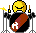# Chords

Like chords but can’t be bothered learning them? Try the following python program:

``````
"""
Generates chords from a single root note.

Route your keyboard through CHORDS.

The chord formulas are consecutive half-steps from
the root note.
"""

from mididings import *

# print flag (set to 1 to print midi notes)
pf = 0

# Chord formulas
chords = (
{
"Chord" : "Major",
"Formula" : [4,3]
},
{
"Chord" : "Minor",
"Formula" : [3,4]
},
{
"Chord" : "Power",
"Formula" : 
},
{
"Formula" : [4,3,7]
},
{
"Chord" : "maj7",
"Formula" : [4,3,4]
},
{
"Chord" : "maj9 (ext)",
"Formula" : [4,3,4,3]
},

)

def Chord(n):
f = chords[n]["Formula"]
r = Pass()
tt = 0
for t in f:
tt = tt + t
r = r // Transpose(tt)
if pf:
r = r >> Print()
return r

scenes={1 : Scene("No chord", Pass())}
for c in range(len(chords)):
scenes[c+2] = Scene(chords[c]["Chord"],Chord(c))

config(
backend='jack-rt',
client_name='CHORDS',
)

run(
scenes,
control = Filter(PROGRAM) >> SceneSwitch(),
pre = ~Filter(PROGRAM),
)

``````

now theres also a tool made by SUVA for renoise